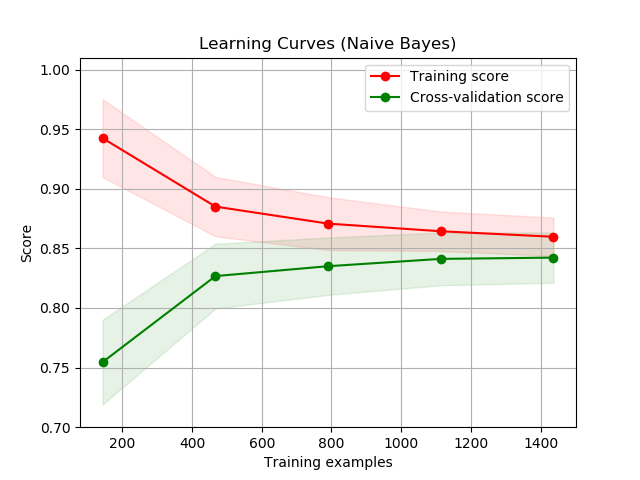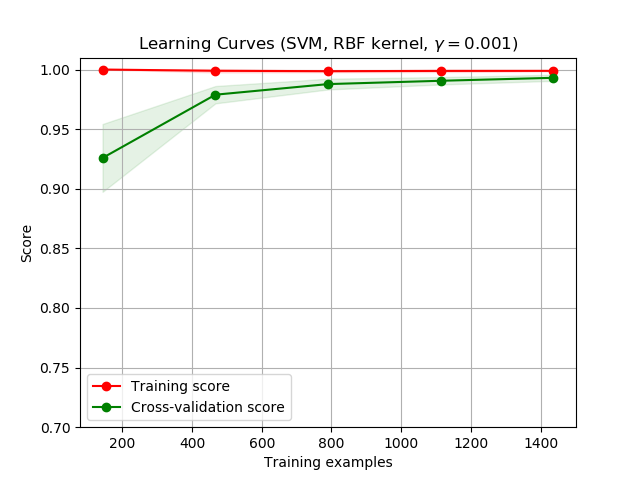# Plotting Learning Curves¶

On the left side the learning curve of a naive Bayes classifier is shown for the digits dataset. Note that the training score and the cross-validation score are both not very good at the end. However, the shape of the curve can be found in more complex datasets very often: the training score is very high at the beginning and decreases and the cross-validation score is very low at the beginning and increases. On the right side we see the learning curve of an SVM with RBF kernel. We can see clearly that the training score is still around the maximum and the validation score could be increased with more training samples.

••print(__doc__)

import numpy as np
import matplotlib.pyplot as plt
from sklearn.naive_bayes import GaussianNB
from sklearn.svm import SVC
from sklearn.model_selection import learning_curve
from sklearn.model_selection import ShuffleSplit

def plot_learning_curve(estimator, title, X, y, ylim=None, cv=None,
n_jobs=None, train_sizes=np.linspace(.1, 1.0, 5)):
"""
Generate a simple plot of the test and training learning curve.

Parameters
----------
estimator : object type that implements the "fit" and "predict" methods
An object of that type which is cloned for each validation.

title : string
Title for the chart.

X : array-like, shape (n_samples, n_features)
Training vector, where n_samples is the number of samples and
n_features is the number of features.

y : array-like, shape (n_samples) or (n_samples, n_features), optional
Target relative to X for classification or regression;
None for unsupervised learning.

ylim : tuple, shape (ymin, ymax), optional
Defines minimum and maximum yvalues plotted.

cv : int, cross-validation generator or an iterable, optional
Determines the cross-validation splitting strategy.
Possible inputs for cv are:
- None, to use the default 3-fold cross-validation,
- integer, to specify the number of folds.
- :term:CV splitter,
- An iterable yielding (train, test) splits as arrays of indices.

For integer/None inputs, if y is binary or multiclass,
:class:StratifiedKFold used. If the estimator is not a classifier
or if y is neither binary nor multiclass, :class:KFold is used.

Refer :ref:User Guide <cross_validation> for the various
cross-validators that can be used here.

n_jobs : int or None, optional (default=None)
Number of jobs to run in parallel.
None means 1 unless in a :obj:joblib.parallel_backend context.
-1 means using all processors. See :term:Glossary <n_jobs>
for more details.

train_sizes : array-like, shape (n_ticks,), dtype float or int
Relative or absolute numbers of training examples that will be used to
generate the learning curve. If the dtype is float, it is regarded as a
fraction of the maximum size of the training set (that is determined
by the selected validation method), i.e. it has to be within (0, 1].
Otherwise it is interpreted as absolute sizes of the training sets.
Note that for classification the number of samples usually have to
be big enough to contain at least one sample from each class.
(default: np.linspace(0.1, 1.0, 5))
"""
plt.figure()
plt.title(title)
if ylim is not None:
plt.ylim(*ylim)
plt.xlabel("Training examples")
plt.ylabel("Score")
train_sizes, train_scores, test_scores = learning_curve(
estimator, X, y, cv=cv, n_jobs=n_jobs, train_sizes=train_sizes)
train_scores_mean = np.mean(train_scores, axis=1)
train_scores_std = np.std(train_scores, axis=1)
test_scores_mean = np.mean(test_scores, axis=1)
test_scores_std = np.std(test_scores, axis=1)
plt.grid()

plt.fill_between(train_sizes, train_scores_mean - train_scores_std,
train_scores_mean + train_scores_std, alpha=0.1,
color="r")
plt.fill_between(train_sizes, test_scores_mean - test_scores_std,
test_scores_mean + test_scores_std, alpha=0.1, color="g")
plt.plot(train_sizes, train_scores_mean, 'o-', color="r",
label="Training score")
plt.plot(train_sizes, test_scores_mean, 'o-', color="g",
label="Cross-validation score")

plt.legend(loc="best")
return plt

X, y = digits.data, digits.target

title = "Learning Curves (Naive Bayes)"
# Cross validation with 100 iterations to get smoother mean test and train
# score curves, each time with 20% data randomly selected as a validation set.
cv = ShuffleSplit(n_splits=100, test_size=0.2, random_state=0)

estimator = GaussianNB()
plot_learning_curve(estimator, title, X, y, ylim=(0.7, 1.01), cv=cv, n_jobs=4)

title = r"Learning Curves (SVM, RBF kernel, $\gamma=0.001$)"
# SVC is more expensive so we do a lower number of CV iterations:
cv = ShuffleSplit(n_splits=10, test_size=0.2, random_state=0)
estimator = SVC(gamma=0.001)
plot_learning_curve(estimator, title, X, y, (0.7, 1.01), cv=cv, n_jobs=4)

plt.show()


Total running time of the script: ( 0 minutes 2.856 seconds)

Gallery generated by Sphinx-Gallery